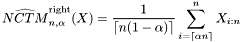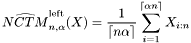#Boost C++ Libraries

...one of the most highly regarded and expertly designed C++ library projects in the world.

This is the documentation for an old version of boost. Click here for the latest Boost documentation.

## Struct template non_coherent_tail_mean_impl

boost::accumulators::impl::non_coherent_tail_mean_impl — Estimation of the (non-coherent) tail mean based on order statistics (for both left and right tails).

## Synopsis

```// In header: <boost/accumulators/statistics/tail_mean.hpp>

template<typename Sample, typename LeftRight>
struct non_coherent_tail_mean_impl {
// types
typedef numeric::functional::average< Sample, std::size_t >::result_type float_type;
typedef float_type                                                       result_type;

// construct/copy/destruct
non_coherent_tail_mean_impl(dont_care);

// public member functions
template<typename Args> result_type result(Args const &) const;
};```

## Description

An estimation of the non-coherent tail meanis given by the mean of thesmallest samples (left tail) or the mean of thelargest samples (right tail),being the total number of samples andthe quantile level:

Equation 1.14.Equation 1.15.It thus requires the caching of at least thesmallest or thelargest samples.

### `non_coherent_tail_mean_impl` public construct/copy/destruct

1. `non_coherent_tail_mean_impl(dont_care);`

### `non_coherent_tail_mean_impl` public member functions

1. `template<typename Args> result_type result(Args const & args) const;`